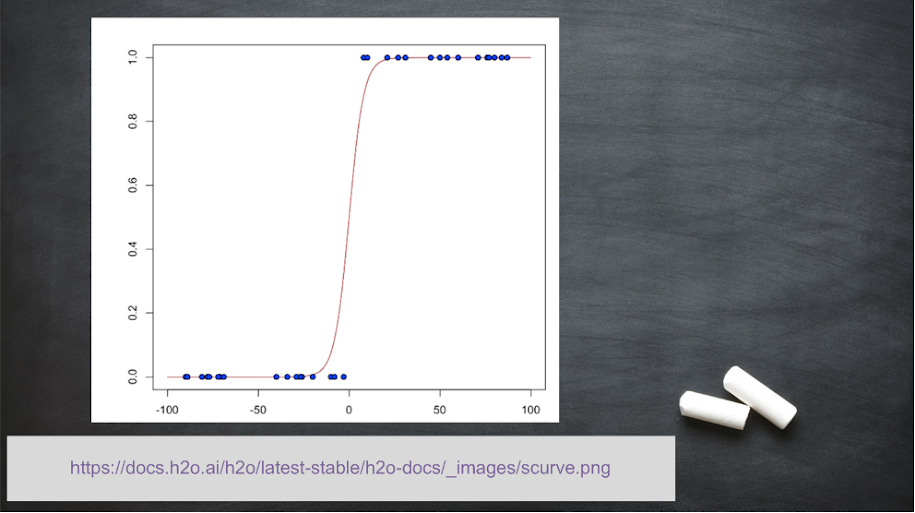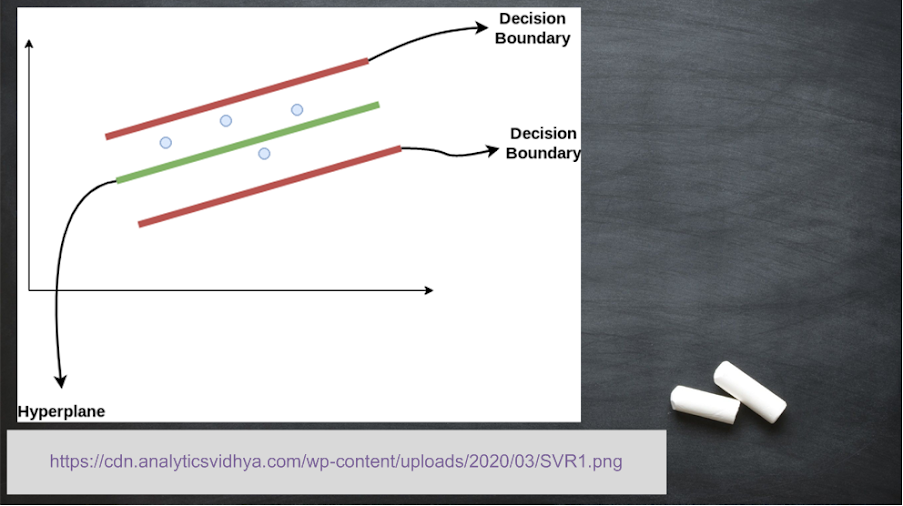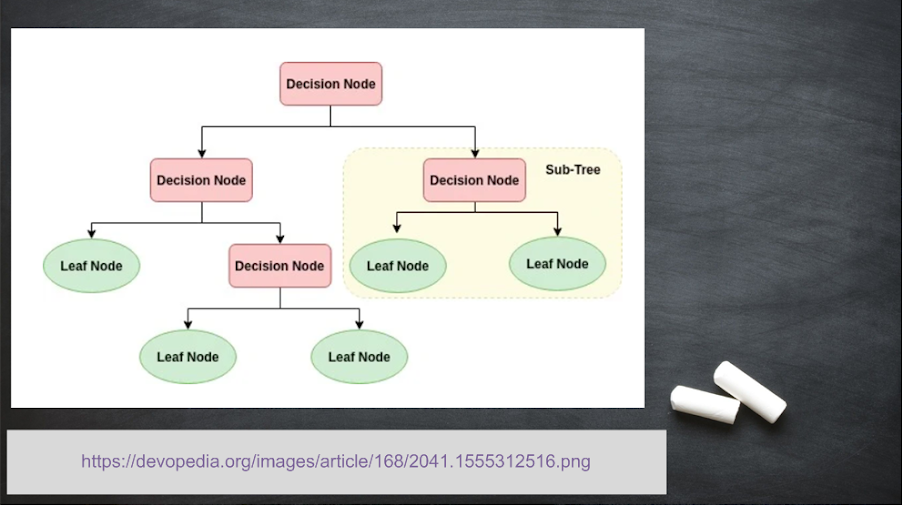So how are you guys!! Its already the fourth month of the year. One-quarter of the year is already gone and we didn’t even notice. You know what else we never noticed – the application of linear techniques to solve non-linear models. Isn’t it strange? Yes, one such regression-based machine learning algorithm is this much strange (not Dr. Strange from Multiverse of Madness 😄. Big Fan 🙌).

### Generalized linear model

In generalized linear regression model, a non-linear function is made fit by using linear combination of inputs. Now what is this “fit”? For now, you can say that a model is said to be “fit” if it works well for testing images as it worked during training period. For instance, a denoised image is to be obtained from noisy image, so the model is fit only if its testing accuracy is equal or close to training accuracy . I know it is complicated, we will surely learn about it in detail in upcoming articles. Key points are as follows:
• Used for non-normal distribution of response variables, such as a response variable which is always positive.### SVM Regression

It is similar to SVM classification technique, but are used for continuous process. Unlike classification technique, hyperplane is not found to separate the data into classes. Instead, it finds the model which is deviated from measured data by a small amount. Key points are as follows:
• Used for data with large number of predictor variables.### Regression Tree

Just like SVM, tree is also modified for prediction task instead of separating data in classes. Key points are as follows:
• Used when predictors are discrete or behave non-linearly.From the next article, we will start with clustering-based machine learning techniques, Please give your feedback on this article of Deep Learning Series and suggestions for future articles in the comment section below 👇.